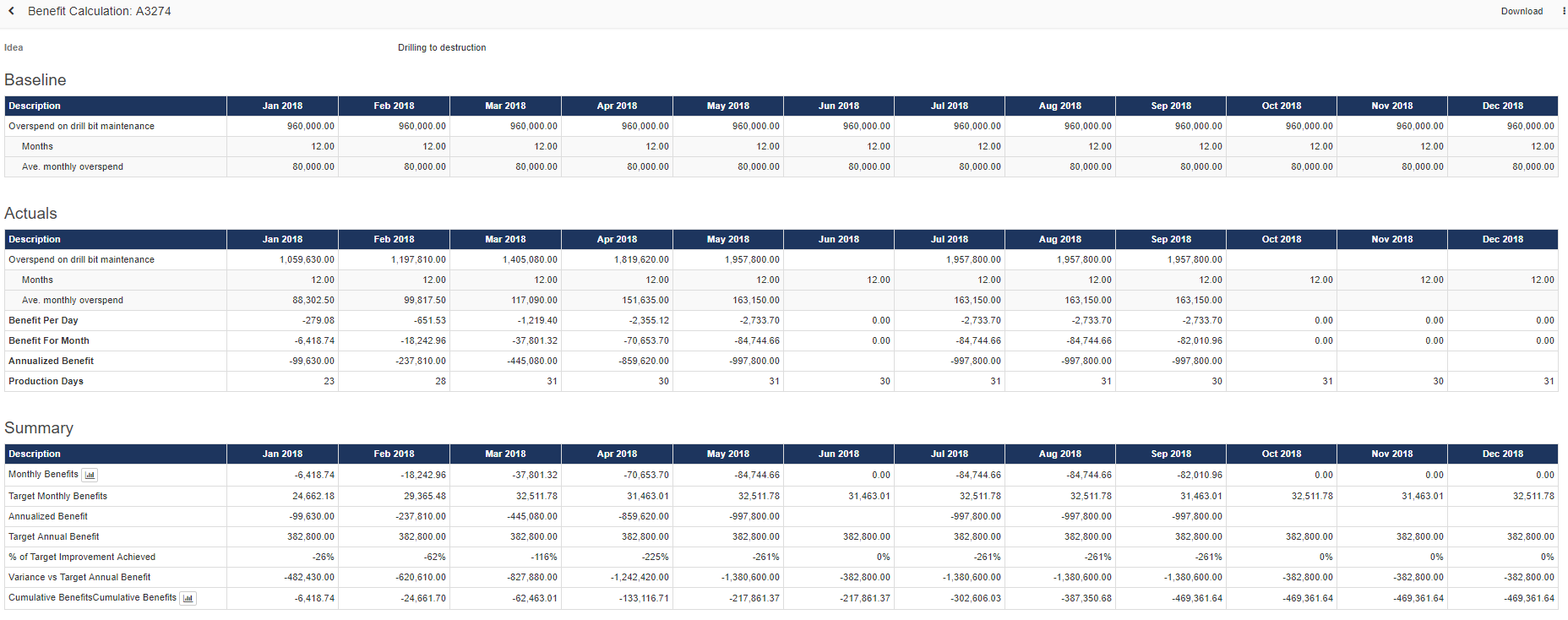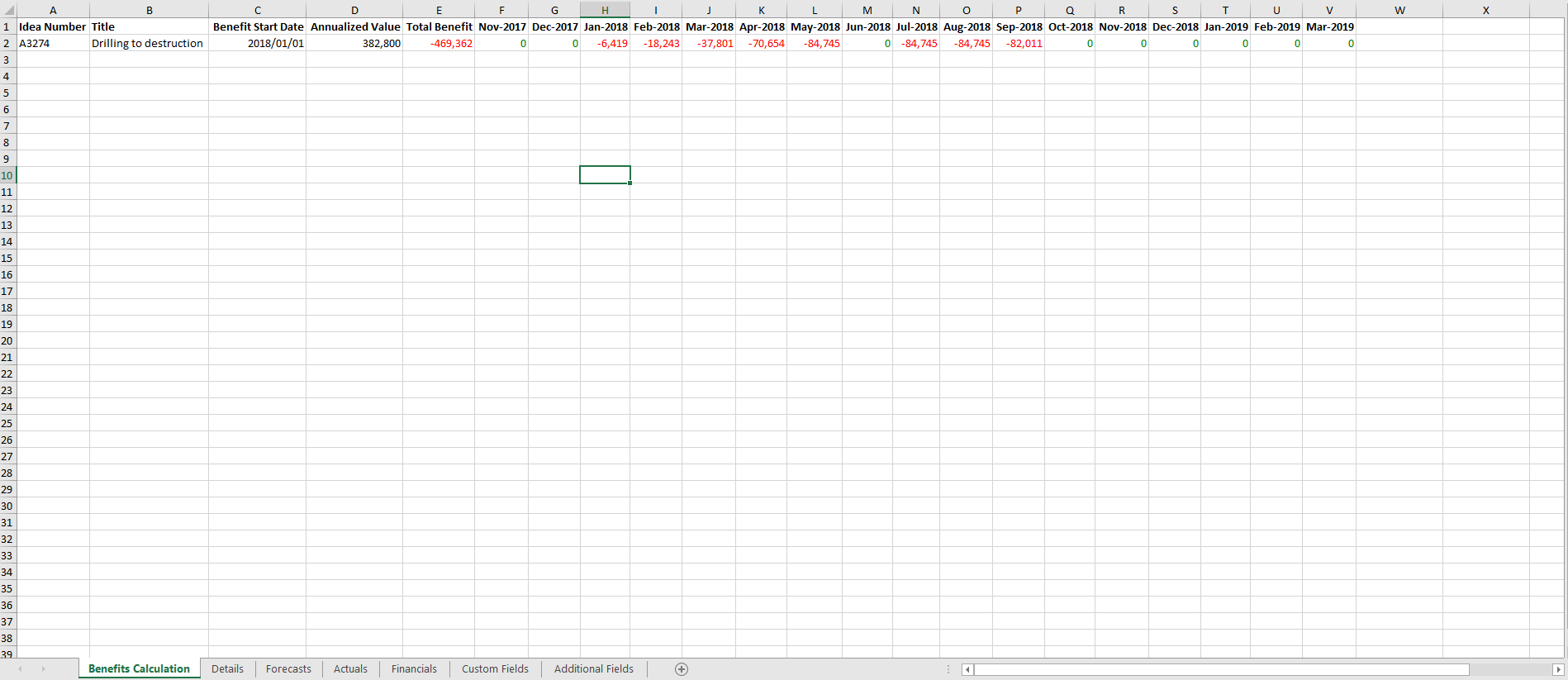Please note the only values that need to be the same between the two reports (Idea Benefit calculation and Cumulative Benefits report) is the actual benefit realized for the month.
• Further note that the benefit realized for a month on the Cumulative Benefits report is affected by the Annualized Benefit Flag.

The details section on the Cumulative Benefits reports download is used to compare the delivered Benefit value with the Target value that was supposed to be delivered for the particular month and then calculate the differences and the variance.

The different calculation is explained below:

• Note this Idea has an Idea Value of 382800 (displayed below as the Target Annual Benefit).The Idea Benefits Calculation section uses the following values to calculate the summary section values. i.e  Benefit for Jan-2018.
• Idea Value(382 800) driven by the VDT (Calculated by applying the formula on the actual and baseline values for the root node)
• The number of days in the month(23), which is relative to each month(as displayed above).
• Total Benefit days (357) (This value is calculated from the default calendar inline with the weekdays excluding site unavailability)
• Idea Annualized benefit value for the month (-99 630) - Which is obtained by subtracting the baseline from the Annualized monthly Actual depending on the trend: ( 960 000.00 - 1 059 630.00) = (-99 630)

Using the values above the Summary is Calculated as follows:

• Monthly Benefit for Jan-2018 = (Annualized benefit / total benefit days * days in the month) = (-99 630 / 357 * 23) = -6 418.739496 = -6 418.74.
• Target Monthly Benefit for Jan-2018 = (Idea Value / total benefit days * days in the months) = (382 800/ 357 * 23) = 24 662.1848 = 24 662.19.
• Annualized Benefit for Jan-2018 = (Annualized benefit from the Actual's) = -99 630
• Target Annual Benefit = Idea Value = 382 800
• % of Target Improvement Achieved = (Annualized benefit Value for the month / idea value) = (-99 630 / 382 800 * 100) = -26.02% = -26%.
• Variance vs Target Annual Benefit = (Annualized Benefit Value - Idea Value) = (-99 630 - 382 800) = -482 430
• Cumulative Benefits = Previous Month Benefit + Current Month Benefit = 0 + (-6 418.74) = -6 418.74.The Details section of the Cumulative Benefits report is calculated as follows:Whilst the Cumulative Benefits report details section is calculated as follows note the Cumulative Benefits report uses the benefit values of the Idea stored on the system.
• This will explicitly display the Target Value that was meant to be achieved for the month of Jan-2018 ( 24 662.184874)
• Then Actual benefit value that was achieved for the month Jan-2018 ( - 6 418.739496).

Using the values above the Details are Calculated as follows:

• The Target obtained from the DB and the Actual that can be cross-referenced with the Idea benefits calculation for the month.
• Variance = (Actual - Target) = ( - 6 418.739496 - 24 662.184874) = - 31 080.92437
• Variance % = ( - 6 418.739496 / 24 662.184874) * 100 = - 26.03 %